# High school + combinatorial number - examples - page 4

1. DiceWe throw 10 times a play dice, what is the probability that the six will fall exactly 4 times?
2. ClassroomOf the 26 pupils in the classroom, 12 boys and 14 girls, four representatives are picked to the odds of being: a) all the girls b) three girls and one boy c) there will be at least two boys
3. Families 2There are 729 families having 6 children each. The probability of a girl is 1/3 and the probability of a boy is 2/3. Find the the number of families having 2 girls and 4 boys.
4. TestThe teacher prepared a test with ten questions. The student has the option to choose one correct answer from the four (A, B, C, D). The student did not get a written exam at all. What is the probability that: a) He answers half correctly. b) He answers.
5. Raffle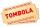There are 200 draws in the raffle, but only 20 of them win. What is the probability of at least 4 winnings for a group of people who have bought 5 tickets together?
6. Three students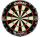Three students independently try to solve the problem. The first student will solve a similar problem with a probability of 0.6, the second student will solve at a probability of 0.55, and the third will solve at a probability 0.04. The problem is resolved
7. Boys and girlsThere are 11 boys and 18 girls in the classroom. Three pupils will answer. What is the probability that two boys will be among them?
8. Diagonals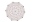What x-gon has 54 diagonals?
9. A pizzaA pizza place offers 14 different toppings. How many different three topping pizzas can you order?
10. Family94 boys are born per 100 girls. Determine the probability that there are two boys in a randomly selected family with three children.
11. A bookA book contains 524 pages. If it is known that a person will select any one page between the pages numbered 125 and 384, find the probability of choosing the page numbered 252 or 253.
12. Combinations 66 purses 9 flaps 12 straps Every combination must include 1 purse, 1 flap and 1 strap. How many possible combinations?
13. Three workplacesHow many ways can we divide nine workers into three workplaces if they need four workers in the first workplace, 3 in the second workplace and 2 in the third?
14. Peaches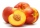There are 20 peaches in the pocket. 3 peaches are rotten. What is the probability that one of the randomly picked two peaches will be just one rotten?
15. Subsets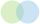How many 19 element's subsets can be made from the 26 element set?
16. Bridge cards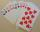How many bridge hands are possible containing 4 spades,6 diamonds, 1 club, and 2 hearts?
17. Cancel fractionsCompress the expression of factorial: (n+6)!/(n+4)!-n!/(n-2)!On the menu are 12 kinds of meal. How many ways can we choose four different meals into the daily menu?
19. Three numbersWe have three different non-zero digits. We will create all 3 digits numbers from them to use all 3 figures in each number. We add all the created numbers, and we get the sum of 1554. What were the numbers?
20. STRESSED wordEach letter in STRESSED is printed on identical cards, one letter per card and assembled in random order. Calculate the probability that the cards spell DESSERTS when assembled.

Do you have an interesting mathematical example that you can't solve it? Enter it, and we can try to solve it.

To this e-mail address, we will reply solution; solved examples are also published here. Please enter e-mail correctly and check whether you don't have a full mailbox.